Need Help?

Subscribe to Circuit

###### \${selected_topic_name}
• Notes

$\begin{array}{l}{\text { a) Use the principle of superposition to find the voltage } v} \\ {\text { b) Find the power dissipated in the } 10 \Omega \text { resistor. }}\end{array}$

Case (1) Use 4A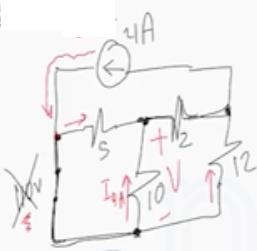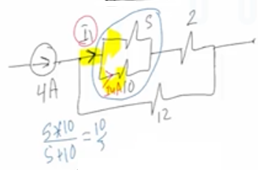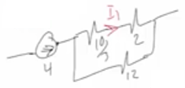$I_{1}=\frac{4 * 12}{12+2+10}=2.77 A$

$I_{4 A}=\frac{2.77*5}{5+10}=0.9233 A$

Case (2) Use 110 V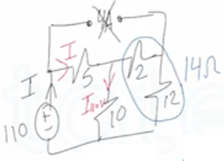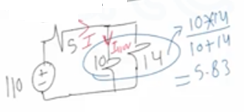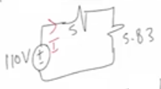$I=\frac{V}{R}=\frac{110}{5+5.83}=10.15 \mathrm{A}$

$I_{110 v}=\frac{10.15 * 14}{14+10}=5.9233 \mathrm{A}$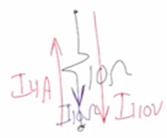$I_{10 \Omega}=I_{110v} -I_{ 4 A}$

$I_{10 \Omega}=5.9233-0.9233$

$I_{10 \Omega}=5 A$

$V=IR=5 * 10=50 v$

$P=I^{2} R=(5)^{2} * 10$

$P=250 \mathrm{w}$

$\text { dissipated }$